# AP Chemistry : Effusion

## Example Questions

### Example Question #1 : Effusion

A mixture of neon gas and argon gas is present in a container (container A). There are equal amounts of both gases in the container. A small pinhole is created in the container, allowing the gases to effuse into an empty container (container B). The effusion time is very brief, and the pinhole is eventually plugged, resulting in a mixture of both gases in both containers.

Which of the following statements is true after the pinhole is plugged?

Container B will contain twice as many neon atoms as argon atoms

The partial pressure for argon is greater than the partial pressure for neon in container A

Container B will contain twice as many argon atoms as neon atoms

Both gases will have equal partial pressures in container A

The partial pressure for argon is greater than the partial pressure for neon in container A

Explanation:

The rate of effusion for two gases can be compared to one another using the following equation: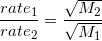Here, the effusion rates are inversely proportional to the square root of the molecular masses of the gases in question. Because the relationship is to the square roots of the molecular masses, we will not observe a 2:1 ratio of effusion for neon compared to argon.

We will, however, see that more neon effuses out of container A compared to the amount of argon because neon is the lighter gas and will thus have a faster effusion rate. As a result, there will be more argon than neon in container A after the pinhole is plugged. This results in argon having a larger partial pressure than neon in container A.

### Example Question #2 : Effusion

A glass box holds equal amounts of hydrogen, nitrogen, oxygen, and bromine. The gases are allowed to exit the container through a tiny hole. Which gas will exit the hole the fastest?

Nitrogen

Oxygen

They all exit at the same rate because the temperature is constant

Bromine

Hydrogen

Hydrogen

Explanation:

At a particular temperature, the average kinetic energy of all gaseous molecules is equal. Since hydrogen gas has the lowest mass out of these gases, it will have the highest average velocity. This means that it will exit out of the tiny hole at a rate faster than the other gases. Conversely, bromine, which has the most mass compared to the other gases, will exit the hole the slowest.

This relationship is mathematically represented in Graham's law: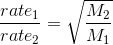As the mass increases, the rate of effusion decreases.

### Example Question #3 : Effusion

Which of the following gases will have the highest rate of effusion?

Sulfur dioxide

Oxygen

Nitrogen

Carbon dioxide

Helium

Helium

Explanation:

The rate of effusion for a gas is inversely proportional to the square-root of its molecular mass (Graham's Law).The gas with the lowest molecular weight will effuse the fastest.

Oxygen: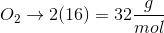Nitrogen: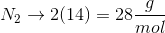Carbon dioxide: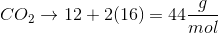Sulfur dioxide: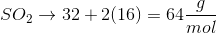Helium: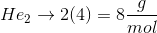The lightest, and therefore fastest, gas is helium.

### Example Question #4 : Effusion

Molecule A has twice the mass of molecule B. A sample of each molecule is released into separate, identical containers. Which compound will have a higher rate of diffusion?

Molecule A

They will have identical rates of diffusion

Molecule B

Molecule A would have a faster initial rate; both molecules would reach an equal final rate

There is not enough information to determine relative rates of diffusion

Molecule B

Explanation:

According to Graham's law, the rate of diffusion of a gas molecule is inversely proportional to the root square of that molecule's mass. Because molecule B has a smaller mass than molecule A, it will have a higher rate of diffusion.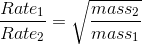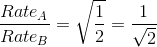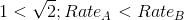Note: this also applies to finding the rate of effusion.

### Example Question #5 : Effusion

A mixture of neon gas and argon gas is present in a container (container A). There are equal amounts of both gases in the container. A small pinhole is created in the container, allowing the gases to effuse into an empty container (container B). The effusion time is very brief, and the pinhole is eventually plugged, resulting in a mixture of both gases in both containers.

Suppose that after the pinhole is plugged, there are 100 argon atoms in container B. Approximately how many neon atoms would you predict to be in container B?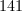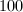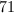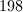Explanation:

We can compare the effusion rates of these gases using the following equation.By calling neon "gas 1" and argon "gas 2," we can compare the effusion rates of the two gases by plugging their molecular masses into the equation.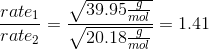This proportion is equal to the rate of neon effusion over the rate of argon effusion, giving the ratio of neon atoms to argon atoms in container B.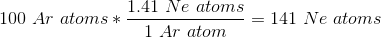As a result, 141 atoms of neon gas will effuse out of the pinhole for every 100 argon gas atoms. Keep in mind that the heavier gas will effuse at a slower rate than the lighter gas; thus, we would expect there to be more neon than argon in container B.

### Example Question #6 : Effusion

A 20cm tube holds two cotton balls, one in each end. The left cotton ball is saturated with undiluted HCl. The right cotton ball is soaked in an undiluted mystery compound. Vapors from the two cotton balls are allowed to mix within the tube.

Let us assume that the two compounds form a precipitate in the tube 6cm to the left of the right cotton ball. What is the molar mass of the mystery compound?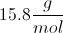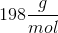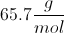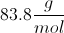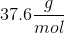Explanation:

This question is notably difficult, as it may not be immediately apparent what concept is being tested. As the vapors of the compounds mix and react, we are able to establish the distance the each vapor has traveled from the cotton ball into the tube in the given amount of time. The tube is 20cm, and the reaction takes place 6cm from the mystery compound cotton ball. From this, we can establish that in an equal amount of time the HCl vapor traveled 14cm and the mystery compound traveled 6cm.

In order to solve this problem, we use Graham's law to compare molar masses to the rates of diffusion of the two gases.Since HCl moved 14cm to the right before interacting with the mystery compound, we know that the mystery compound moved only 6cm to the left. As a result, the diffusion ratio is 2.33.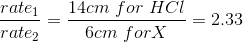Now, we need to find the square root of the inversed molar masses, which equals this diffusion ratio.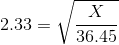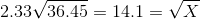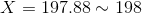So, the molar mass of the mystery compound is 198 grams per mole. This makes sense, because larger gases will move more slowly compared to lighter gases.

### Example Question #7 : Effusion

Which of the following gases has the highest rate of effusion?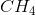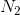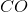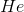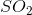Explanation:

The rate of effusion of a gas is inversely proportional to the square root of the molecular weight of the gas.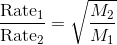The lighter a gas is, the faster it will effuse; the heavier a gas is, the slower it will effuse.

Of all the choices, helium (He) has the lowest molecular weight (atomic weight in this case), so it will have the highest rate of effusion.

### Example Question #8 : Effusion

Gas A has a molar mass that istimes greater than that of Gas B. Which of these gases would be expected to effuse through a small hole faster? By how much?

Gas A effusestimes faster than Gas B

Gas B effusestimes faster than Gas A

Gas A effusestimes faster than Gas B

Gas B effusestimes faster than Gas A

Gas B effusestimes faster than Gas A

Explanation:

In order to answer this question, let's start by considering what effusion is and what things affect it. Effusion is the movement of a gas through a tiny hole that separates two different spaces. Because the gas particles move around in random directions with an average speed that is dependent on the temperature of the sample, lighter gas particle will move faster than heavier gas particles. This is because at a given temperature, all gas particles in a sample will have the same average kinetic energy. Consequently, we would expect gas particles with a higher molar mass to effuse more slowly than gases with a lower molar mass. This means that Gas B should effuse faster than Gas A.

The next step is to actually calculate how much greater Gas B effuses compared to Gas A. To do this, we'll need to use the following equation: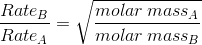Since we know that Gas A istimes heavier than Gas B, we can plug this into the equation to solve for the ratio of Gas B's rate of effusion to that of Gas A.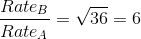Therefore, Gas B effusestimes faster than Gas A.

### Example Question #9 : Effusion

How much faster/slower the rate of effusion for oxygen gas compared to hydrogen gas?

4 times faster

2 times slower

16 times slower

2 times faster

4 times slower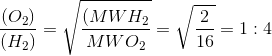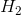and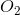must be used because they exist as bimolecular molecules. The correct answer is that Oxygen gas will effuse 4 times slower than hydrogen gas.